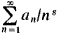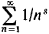# Dirichlet Series

Also found in: Wikipedia.

## Dirichlet series

[‚dē·rē′klā ‚sir·ēz]
(mathematics)
A series whose n th term is a complex number divided by n to the z th power.
McGraw-Hill Dictionary of Scientific & Technical Terms, 6E, Copyright © 2003 by The McGraw-Hill Companies, Inc.
The following article is from The Great Soviet Encyclopedia (1979). It might be outdated or ideologically biased.

## Dirichlet Series

(named for P. G. L. Dirichlet), series of the formwhere the an are constants and s = σ + it is a complex variable. For example, the seriesrepresents the zeta function for σ > 1. The theory of Dirichlet series originally arose under the strong influence of analytic number theory. Eventually it developed into an extensive branch in the theory of analytic functions.

The Great Soviet Encyclopedia, 3rd Edition (1970-1979). © 2010 The Gale Group, Inc. All rights reserved.
References in periodicals archive ?
The periodic zeta-function [zeta](s; a) is defined, for [sigma] > 1, by the Dirichlet series
In 2001, Navas  obtained analytic continuation of the Fibonacci Dirichlet series [[zeta].sub.F] (s).
Among the topics are quadratic points of classical modular curves, p-adic point counting on singular super-elliptic curves, a vanishing criterion for Dirichlet series with periodic coefficients, the Sato-Tate conjecture for a Picard curve with a complex multiplication, arithmetic twists with abelian extensions, and transcendental numbers with special values of Dirichlet series.
where [B.sub.0](x) is given and [a.sub.0] = [[integral].sup.1.sub.0] [B.sub.0](x)dx; they used these generalized Bernoulli polynomials to derive formulas of certain Dirichlet series.
In general the theory of polynomials and multilinear operators between normed spaces has its importance in different areas of Mathematics, from Number Theory, or Dirichlet series, to Functional Analysis.
The kernel function can be expressed in Dirichlet Series form :
Gunnells, Metaplectic ice, in Multiple Dirichlet Series, L-Functions, and Automorphic Forms, D.
where the Dirichlet series G(s); = [[summation].sup.[infinity].sub.n = 1] g(n)/[n.sup.s][infinity] is absolutely convergent for Rs = [sigma] > 1/6.
Widder (1898-1990) drew material from a course of lectures that he gave now and then at Harvard on Dirichlet series and Laplace integrals, to explain the Laplace transform for students who have a fundamental knowledge of analysis, though proofs are included for those with only a little such knowledge.
In this note, we will show that the set of functions [[xi].sub.[alpha]] ([alpha] [member of] C) forms an Abelian group with the Dirichlet series multiplication followed by a number of applications.
However, the formula may be used to construct twin prime Dirichlet series. Such a method is applied here to twin primes p,p' = p + 2D for odd D > 0 first and then even D > 0.

Site: Follow: Share:
Open / Close